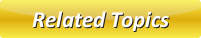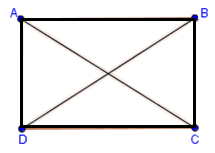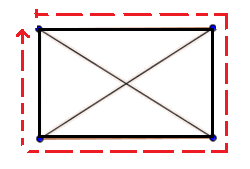# Perimeter of Rectangle

A rectangle can be explained as a 4-sided quadrilateral which contains equal opposite sides. In a rectangle, the opposite sides are always parallel to each other and commonly classified as equiangular quadrilateral. As the rectangle has no equal adjacent sides, we always get 2 different measurements. In the below image, the corners are A, B, C and D. The sides of this rectangle are AB, BC, CD and AD. Finally, the diagonals are AC and BD.�M

## How to Calculate the Perimeter of a Rectangle?

Perimeter of a rectangle is nothing but the overall distance outside the rectangle as shown in the below image:## Steps to Calculate the Perimeter of a Rectangle

1. Check the length and the width for the given rectangle
2. By short, we'll call the length as 'l' and width as 'w'.
3. Apply the formula, 2(l+w) or just add l + w + l + w and you've got the perimeter for the rectangle.

## Perimeter of a Rectangle Problems

Problem 1: A rectangle is 6m long and 5 m wide. What is its perimeter?

Solution: Length, l = 6m.

Width, w = 5m.

Perimeter of the rectangle = 2(l+w) = 2(6+5) = 2 x 11 = 22 m.

Problem 2: A rectangle is 5cm long and its perimeter is 18cm. What is its width?

Solution: Length, l = 5cm.

Width, w say.

Perimeter of the rectangle = 18 cm.

Perimeter of the rectangle = 2(l+w)= 2l + 2w = 2(5) + 2w = 10 + 2w

Thus,   10 + 2w = 18

2w = 18 - 10 = 8

w = 4

Thus the width of the rectangle is 4 cm.

Problem 3: In a rectangle's length is 5x + 2 and its width is 5x - 2. If its Perimeter is 40 cm, what is the value of x?

Solution:  Length, l = 5x + 2.

Width, w = 5x - 2.

The Perimeter of the rectangle = 40 cm. ......(1)

Also by the definition of Perimeter of rectangle,

Perimeter of rectangle = 2(l*w) = 2[(5x + 2)+(5x - 2)]  .....(2)

Equating (1) and (2),

2[(5x + 2)+(5x - 2)] = 40

(5x + 2)+(5x - 2) = 40/2 = 20

10x = 20

x = 20/10

x = 2

Latest Articles

Average Acceleration Calculator

Average acceleration is the object's change in speed for a specific given time period. ...

Free Fall Calculator

When an object falls into the ground due to planet's own gravitational force is known a...

Permutation

In Mathematics, the permutation can be explained as the arrangement of objects in a particular order. It is an ordered...

Perimeter of Rectangle

A rectangle can be explained as a 4-sided quadrilateral which contains equal opposite sides. In a rectangle

Perimeter of Triangle

A three sided polygon which has three vertices and three angles is called a triangle. Equilateral triangle...